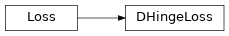DHingeLoss¶

Inheritance Diagramclass ashpy.keras.losses.DHingeLoss[source]

Bases: tensorflow.python.keras.losses.Loss

Discriminator Hinge Loss as Keras Metric.

See Geometric GAN 1 for more details.

The Discriminator Hinge loss is the hinge version of the adversarial loss. The Hinge loss is defined as:

$L_{\text{hinge}} = \max(0, 1 -t y)$

where y is the Discriminator output and t is the target class (+1 or -1 in the case of binary classification).

For the case of GANs:

$L_{D_{\text{hinge}}} = - \mathbb{E}_{(x,y) \sim p_data} [ \min(0, -1+D(x,y)) ] - \mathbb{E}_{x \sim p_x, y \sim p_data} [ \min(0, -1 - D(G(z),y)) ]$
1

Geometric GAN https://arxiv.org/abs/1705.02894

Methods

 __init__() Initialize the Loss. call(d_real, d_fake) Compute the hinge loss.

Attributes

 reduction Return the current reduction for this type of loss.
__init__()[source]

Initialize the Loss.

Return type

None

call(d_real, d_fake)[source]

Compute the hinge loss.

Return type

Tensor

property reduction

Return the current reduction for this type of loss.

Return type

ReductionV2